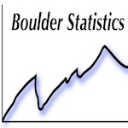Choose Language Hide Translation Bar
HighlightedSuper UserJoined:

Feb 10, 2013

## Custom function over limited x range?

I am adding a function to a graphic (e.g., fit y- by- x) via "Customize Graph": Y Function(_function_of_x_, x);

Can I limit the range of the x's that go into the function such that the function only plots over part of the range of the plot?  So if my x-axis spans from 0 to 100 I only want my function to plot say from x=  50 to 100.

1 ACCEPTED SOLUTION

Accepted Solutions
Solution

## Re: Custom function over limited x range?

You should be able to add an IF function around your "_function_of_x_". In other words:

Y Function( If( 50<x<100, _function_of_x_ ), x );

If x is not in that range, no value is produced.

3 REPLIES 3
Solution

## Re: Custom function over limited x range?

You should be able to add an IF function around your "_function_of_x_". In other words:

Y Function( If( 50<x<100, _function_of_x_ ), x );

If x is not in that range, no value is produced.

## Re: Custom function over limited x range?

Try the optional Min() and Max() arguments.

dt = Open("\$SAMPLE_DATA/Big Class.jmp");

biv = dt << Bivariate(Y(:Weight), X(:Height));

Report(biv)[Framebox(1)] << add graphics script(Y Function(3 * x - 90, x, Min(60), Max(70)));Super UserJoined:

Feb 10, 2013

## Re: Custom function over limited x range?

Excellent, both methods work as does

Y Function (If ( (x>50) & (x<100), function_of_x,) x);

I think I like the Min/Max solution best as the parentheses structure is easier to follow (i.e., less prone to error).

Y Function (function_of_x, x, Min(a), Max(b));